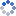加载中…小小四与九阿哥
• 博客等级：• 博客积分：0
• 博客访问：275,454
• 关注人气：69
• 获赠金笔：0支
• 赠出金笔：0支
• 荣誉徽章：

## 如何把两列数据中不同的数值提取（展现\返回）出来？(2013-11-02 13:00:21)

### 高手2.先选中C2至C6，接着直接按“=”键，输入公式：IF(ROW()<=SUMPRODUCT(--(COUNTIF(B\$2:B\$6,A\$1:A\$7)=0)),INDEX(A:A,SMALL(IF(COUNTIF(B\$2:B\$6,A\$1:A\$7),4^8,ROW(\$1:\$7)),ROW())),INDEX(B:B,SMALL(IF(COUNTIF(A\$1:A\$7,B\$2:B\$6),4^8,ROW(\$2:\$6)),ROW()-SUMPRODUCT(--(COUNTIF(B\$2:B\$6,A\$1:A\$7)=0)))))&""0